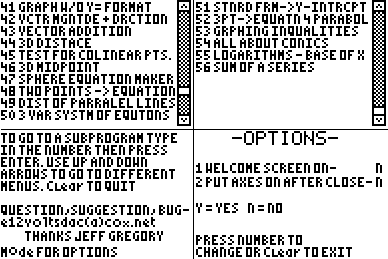Basics Archives Community Services ProgrammingHome :: Archives :: File Archives :: Ultimate Math Suite!Ultimate Math Suite!

FILE INFORMATION

ultimatemathsuite.zip
 Filename ultimatemathsuite.zip (Download) Title Ultimate Math Suite! Description Math from pre-algebra through calculus. Includes: complete the square, direct/indirect variation, number of solutions, midpoint, prime numbers, Pythagorean theorem, quadratic formula, slope, distance, vertex, area, volume, surface area, y intercept, Fibonacci sequence, circumference, average, simplified square roots, proportions, system of equations, distance of point to line, types of quadrilaterals, scientific notation, a point of an isosceles trapezoid, Pythagorean triples, law of sines/cosines, DMS, geometric mean, circle equation maker, lateral area, apothem finder, point-slope to Y-intercept, frustums, graphing without Y= format, graphing < and >, vector magnitude and direction, vector addition, and 3D distance, test for collinear points, 3D midpoint, sphere equation maker, distance of parallel lines, modulus, 3 variable system of equations, standard form to y intercept, cramers rule, 3 points to equation for parabola, graphing inequalities, All about conics (equation makers, details about, and etc.), logarithms of X base, iterations, sequences, and sum of sequences, Cramer's Rule for a system of 3 equations Author Josh Lien (calcpro95@yahoo.com) Category TI-83/84 Plus BASIC Math Programs (Suites) File Size 9,639 bytes File Date and Time Mon Aug 11 04:11:45 2008 Documentation Included? No

SCREEN SHOTSREVIEWS

There are no reviews for this file.

LEAVE FEEDBACK

Questions, comments, and problems regarding the file itself should be sent directly to the author(s) listed above.

Report inappropriate or miscategorized file (requires an account; or you may email us directly)